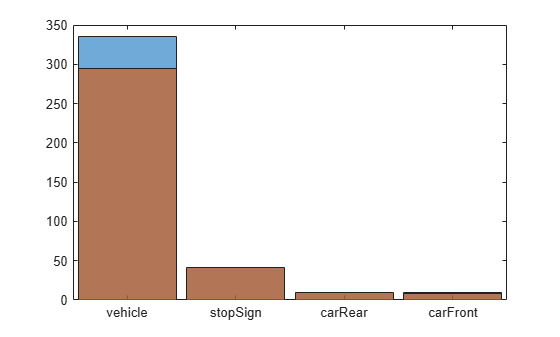Documentation

# countEachLabel

Count occurrence of pixel or box labels

## Syntax

``counts = countEachLabel(ds)``

## Description

example

````counts = countEachLabel(ds)` returns a table containing information about the pixel or box labels and count for the input datastore, `ds`.```

## Examples

collapse all

Load a table that contains bounding boxes with labels for vehicles.

`load('vehicleTrainingData.mat');`

Load a table that contains bounding boxes with labels for stop signs and cars.

`load('stopSignsAndCars.mat');`

Combine ground truth boxes and labels, excluding the image filenames in the first column.

```vehiclesTbl = vehicleTrainingData(:,2:end); stopSignsTbl = stopSignsAndCars(:,2:end);```

Create a boxLabelDatastore using 2 tables, one with vehicle label data and the other with stop signs label data.

```blds = boxLabelDatastore(vehiclesTbl,stopSignsTbl); tbl = countEachLabel(blds)```
```tbl=4×3 table Label Count ImageCount ________ _____ __________ vehicle 336 295 stopSign 42 41 carRear 10 9 carFront 9 8 ```

Create a histogram plot using the labels and the respective label counts.

`histogram('Categories',tbl.Label,'BinCounts',tbl.Count);`

Create another histogram overlaying the respective image counts.

```hold on; histogram('Categories',tbl.Label,'BinCounts',tbl.ImageCount);```Set the location of image and pixel label data.

```dataDir = fullfile(toolboxdir('vision'),'visiondata'); imDir = fullfile(dataDir,'building'); pxDir = fullfile(dataDir,'buildingPixelLabels');```

Create a pixel label image datastore using the ground truth images in `imds` and the pixel labeled images in `pxds`.

```imds = imageDatastore(imDir); classNames = ["sky" "grass" "building" "sidewalk"]; pixelLabelID = [1 2 3 4]; pxds = pixelLabelDatastore(pxDir,classNames,pixelLabelID); pximds = pixelLabelImageDatastore(imds,pxds);```

Tabulate pixel label counts in dataset.

`tbl = countEachLabel(pximds)`
```tbl=4×3 table Name PixelCount ImagePixelCount ____________ __________ _______________ {'sky' } 3.1485e+05 1.536e+06 {'grass' } 1.5979e+05 1.536e+06 {'building'} 1.0312e+06 1.536e+06 {'sidewalk'} 25313 9.216e+05 ```

Balance classes using uniform prior weighting.

```prior = 1/numel(classNames); uniformClassWeights = prior./tbl.PixelCount```
```uniformClassWeights = 4×1 10-5 × 0.0794 0.1565 0.0242 0.9876 ```

Balance classes using inverse frequency weighting.

```totalNumberOfPixels = sum(tbl.PixelCount); frequency = tbl.PixelCount / totalNumberOfPixels; invFreqClassWeights = 1./frequency```
```invFreqClassWeights = 4×1 4.8632 9.5827 1.4848 60.4900 ```

Balance classes using median frequency weighting.

`freq = tbl.PixelCount ./ tbl.ImagePixelCount`
```freq = 4×1 0.2050 0.1040 0.6714 0.0275 ```
`medFreqClassWeights = median(freq) ./ freq`
```medFreqClassWeights = 4×1 0.7538 1.4852 0.2301 5.6252 ```

Pass the class weights using median frequency weighting to the pixel classification layer.

```layer = pixelClassificationLayer('Classes',tbl.Name, ... 'ClassWeights', medFreqClassWeights)```
```layer = PixelClassificationLayer with properties: Name: '' Classes: [sky grass building sidewalk] ClassWeights: [4x1 double] OutputSize: 'auto' Hyperparameters LossFunction: 'crossentropyex' ```

## Input Arguments

collapse all

Datastore with labeled data for training a semantic segmentation network or an object detection network, specified as a `pixelLabelDatastore`, `pixelLabelImageDatastore`, or `boxLabelDatastore` object.

## Output Arguments

collapse all

Label information, returned as a table. The labeled data table contain three variables.

For `pixelLabelDatastore` and `pixelLabelImageDatastore` inputs, the `counts` output contains:

Pixel Count VariablesDescription
`Name`Pixel label class name
`PixelCount`Number of pixels in class
`ImagePixelCount`Total number of pixels in images that had an instance of the class

For `boxLabelDatastore` inputs, the `counts` output table contains:

Box Count VariablesDescription
`Label`Box label class name
`Count`Total number of labels of the class across all images
`ImageCount`Total number of images that contain one or more instances of the class

## Tips

The output of `countEachLabel` can be used to calculate class weights for class balancing. For example, for labeled pixel data information in `tbl`:

• Uniform class balancing weights each class such that each contains a uniform prior probability:

```numClasses = height(tbl) prior = 1/numClasses; classWeights = prior./tbl.PixelCount```

• Inverse frequency balancing weights each class such that underrepresented classes are given higher weight:

```totalNumberOfPixels = sum(tbl.PixelCount) frequency = tbl.PixelCount / totalNumberOfPixels; classWeights = 1./frequency```

• Median frequency balancing weights each class using the median frequency. The weight for each class is defined as median(imageFreq)/imageFreq(c), where imageFreq(c) represents the number of pixels of the class divided by the total number of pixels in images that had an instance of the class (c):

```imageFreq = tbl.PixelCount ./ tbl.ImagePixelCount classWeights = median(imageFreq) ./ imageFreq ```
The calculated class weights can be passed to the `pixelClassificationLayer`.